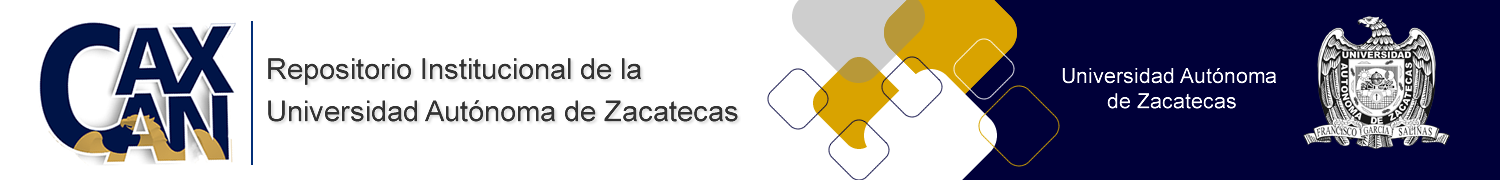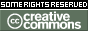Por favor, use este identificador para citar o enlazar este ítem: `http://ricaxcan.uaz.edu.mx/jspui/handle/20.500.11845/2045`
 Título : Post-Newtonian limit: second-order Jefimenko equations Autor : 1204850000-0002-1478-7946 Fecha de publicación : 23-jul-2020 Editorial : Springer Resumen : The purpose of this paper is to get second-order gravitational equations, a correction made to Jefimenko’s linear gravitational equations. These linear equations were first proposed by Oliver Heaviside in , making an analogy between the laws of electromagnetism and gravitation. To achieve our goal, we will use perturbation methods on Einstein field equations. It should be emphasized that the resulting system of equations can also be derived from Logunov’s non-linear gravitational equations, but with different physical interpretation, for while in the former gravitation is considered as a deformation of space-time as we can see in [2–5], in the latter gravitation is considered as a physical tensor field in the Minkowski space-time (as in [6–8]). In Jefimenko’s theory of gravitation, exposed in [9,10], there are two kinds of gravitational fields, the ordinary gravitational field, due to the presence of masses, at rest, or in motion and other field called Heaviside field due to and acts only on moving masses. The Heaviside field is known in general relativity as Lense-Thirring effect or gravitomagnetism (The Heaviside field is the gravitational analogous of the magnetic field in the electromagnetic theory, its existence was proved employing the Gravity Probe B launched by NASA (See, for example, [11,12]). It is a type of gravitational induction), interpreted as a distortion of space-time due to the motion of mass distributions, (see, for example [13,14]). Here, we will present our second-order Jefimenko equations for gravitation and its solutions. URI : http://ricaxcan.uaz.edu.mx/jspui/handle/20.500.11845/2045 ISSN : 1434-60441434-6052 Otros identificadores : info:eu-repo/semantics/publishedVersion Aparece en las colecciones: *Documentos Académicos*-- UA Cien. y Tec. de la Luz y la Mat. (LUMAT)

Ficheros en este ítem:
Fichero Descripción Tamaño Formato
Este ítem está sujeto a una licencia Creative Commons Licencia Creative Commons